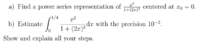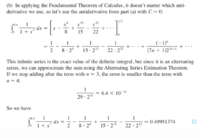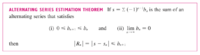# Evaluate a Power Series Integral by Alternating Series Estimation Theorem

#### Mampac

##### New memberHello,

I managed to find the power series form for the function -- separated x2, rewrote the denominator as 1 - (-2x)3, got a power series with n running from 0 to infinity with the term (-1)n23x3n. I pulled out the 23 as 8 and got 8x2 times the power series.

For the integral, I integrated the power series by term-by-term integration and evaluated from 0 to 1/4.

Now I got some issues in b: my question is, for the remainder |R| <= an + 1 <= 10-2, should I include the 8x2 in my computations?

I proceeded to write that an+1-1 => 100, and plugged in ns, starting from 1, inside the an+1-1.

What to do with 8x2 then? Maybe I shouldn't've pulled it out of the series, or maybe after I find the Sn (it turned out S1 suffices since the condition was met at n = 1) I have to do additional computations?

I mean it seems like I found a value but it looks extremely suspicious.

Thank you in advance!

#### lex

##### Full Member
with the term (-1)n23x3n. I pulled out the 23 as 8 and got 8x2 times the power series.
The term isn't correct. Try it again.

The question is all rather silly anyway, as it can easily be integrated directly.
Also, even if you integrate the power series, it is easy to sum exactly.

Last edited:

#### Subhotosh Khan

##### Super Moderator
Staff member
The term isn't correct. Try it again.

The question is all rather silly anyway, as it can easily be integrated directly.
Also, even if you integrate the power series, it is easy to sum exactly.
But that gives a check on the answer gotten by hard way....

#### lex

##### Full Member
But that gives a check on the answer gotten by hard way....
Better to check the work than the answer!

#### Mampac

##### New member
The term isn't correct. Try it again.

The question is all rather silly anyway, as it can easily be integrated directly.
Also, even if you integrate the power series, it is easy to sum exactly.
I rewrite it as 1/(1 – (-2³x³)), then everything in parentheses gets to the power of n. The only thing incorrect by my previous computation seems to be (-1)ⁿ which should be to the power of 3n instead. Does that change much, tho? I'd get it had it been even (then I'd cancel it) but...
Also I didn't quite get whether I should keep the 8x² inside the sum when evaluating by giving n values.

#### nasi112

##### Full Member
Try this power series

$$\displaystyle x^2\sum_{k = 0}^{\infty} (-1)^k (2x)^{3k}$$

•lex and Mampac

#### Mampac

##### New member
Try this power series

$$\displaystyle x^2\sum_{k = 0}^{\infty} (-1)^k (2x)^{3k}$$
omg, i finally spotted my mistake. thanks!

•lex and nasi112

#### lex

##### Full Member
@Mampac
Sorry for the delay in getting back. I see nasi112 has straightened things out.
I'll assume you are ok then.

•nasi112

#### nasi112

##### Full Member
@Mampac
Sorry for the delay in getting back. I see nasi112 has straightened things out.
I'll assume you are ok then.
I have read your file. Brilliant lex, i think that it is better to upload it again so the OP can get the idea of how to use the estimation formula.

•lex

#### lex

##### Full Member
I have read your file. Brilliant lex, i think that it is better to upload it again so the OP can get the idea of how to use the estimation formula.
I don't know how brilliant it is!
It uses a more general method of estimation. There is a very simple method (which the OP clearly knows) for estimating the error in this type of alternating series: Alternating Series Estimation Theorem e.g. here: https://www.kristakingmath.com/blog/alternating-series-estimation-theorem
I thought my file would only confuse the issue - given that they clearly are able to use the simpler method. I thought, why potentially create a problem where there is none?

•nasi112 and Subhotosh Khan

#### nasi112

##### Full Member
I don't know how brilliant it is!
It uses a more general method of estimation. There is a very simple method (which the OP clearly knows) for estimating the error in this type of alternating series: Alternating Series Estimation Theorem e.g. here: https://www.kristakingmath.com/blog/alternating-series-estimation-theorem
I thought my file would only confuse the issue - given that they clearly are able to use the simpler method. I thought, why potentially create a problem where there is none?
That website is for Kristina. I know her and I admit she is a great tutor. I myself referred to her a lot of times, but in the page you sent she explained a lot of things, and I don't think that in a test you will be able to do all these steps.

Instead, I am going to explain a short method and I want you to tell me if I have any errors.

$$\displaystyle x$$ converges in this interval $$\displaystyle (\frac{-1}{2},\frac{1}{2})$$ and the maximum limit of the integral is $$\displaystyle \frac{1}{4}$$, so we are in the safe side.

with $$\displaystyle x = \frac{1}{4}$$, we have

$$\displaystyle a_{k + 1} = \frac{1}{8^{k+1}} \leq 10^{-2}$$

$$\displaystyle 8^{k+1} \geq 100$$

$$\displaystyle k + 1 = \log_8 100$$

$$\displaystyle k = \log_8 100 - 1 \approx 1.21461873$$

this means that with $$\displaystyle k \geq 2$$, we guarantee an error of no more than $$\displaystyle 10^{-2}$$

and here $$\displaystyle k$$ is the number of terms needed

Now, let us compare the actual integral with the approximation we have just found

$$\displaystyle \int_0^{\frac{1}{4}} \frac{x^2}{1 + (2x)^3} = 0.0049076$$

while

$$\displaystyle S_2 = \frac{1}{3}\left(\frac{1}{4}\right)^3 - \frac{8}{6}\left(\frac{1}{4}\right)^6 = \frac{5}{1024} = 0.0048828$$

our error here is $$\displaystyle 1 - \frac{0.0048828}{0.0049076} = 0.00505 < 0.01$$ which proves we are in the required range of precision

#### lex

##### Full Member
Instead, I am going to explain a short method and I want you to tell me if I have any errors.
\displaystyle \text{ }\\ \begin{align*} \text{The series is } \hspace1ex \sum_{k=0}^{\infty} & \frac{(-8)^{k}\left(\frac{1}{4}\right)^{3k+3}}{3k+3} \\ = \frac{1}{64} \sum_{k=0}^{\infty} & \frac{\left(-\frac{1}{8}\right)^{k}}{3k+3}\\ \end{align*}

Clearly this is an alternating series, with $$\displaystyle a_{n+1}≤a_n, |a_n| \to 0$$ as $$\displaystyle n \to \infty$$
$$\displaystyle \therefore$$ by the alternating series test, the series converges
and by the alternating series remainder theorem, the remainder after summing to $$\displaystyle a_n$$ is: $$\displaystyle |R_n|≤a_{n+1}$$

In our case $$\displaystyle |R_n|≤\frac{1}{64}\frac{\left(-\frac{1}{8}\right)^{n+1}}{3n+6}$$

Substituting n=0, we get $$\displaystyle |R_0|≤\frac{1}{3072}$$ (<0.01)

Therefore our estimate is just a single term in the series (with k=0): $$\displaystyle \frac{1}{64}\cdot\frac{1}{3}=\frac{1}{192}$$

A few notes:
1. In the link, I didn't even see the video. I was only trying to show a statement of the Alternating Series Remainder Theorem - i.e. just the text.
2. As seen above, once you have shown that the series is alternating and converges, the magnitude of the remainder term is immediately ≤ $$\displaystyle a_{n+1}$$
so there's no great work involved.
3. Make sure you use the whole term of the series, including the fraction outside and the bottom line inside the sigma. They are all involved in the error in your approximation (and in this case help a lot in reducing the error). That makes your 'calculation' of when the error is less than 0.01, using logs etc., too complicated. However, even though it seems unsophisticated, you simply substitute in values of n, to try to get the error to the acceptable size. It's easy, direct and quick.

Last edited:
•nasi112

#### nasi112

##### Full Member
lex, you applied integration on the series, then you have done the test, but the alternating series estimation is done on the original series + 1

which means just take $$\displaystyle a_n$$ and make it $$\displaystyle a_{n+1}$$ and directly compare it with the precision required as I did.

I don't know if taking integration is another way to solve, but according to my calculus book, the process is like what I did, so we need at least two terms to guarantee the required precision, not only one.

#### lex

##### Full Member
lex, you applied integration on the series, then you have done the test, but the alternating series estimation is done on the original series + 1
For some reason I missed this post.

The alternating series remainder theorem is used on an alternating series which converges etc... (see above).
(It doesn't matter that you were working with another alternating series earlier).
In any case the original series was $$\displaystyle \sum_{k=0}^{\infty} (-8)^k x^{3k+2}$$
Btw what is the name of your calculus book?

Last edited:
•nasi112

#### nasi112

##### Full Member
For some reason I missed this post.

The alternating series remainder theorem is used on an alternating series which converges etc... (see above).
(It doesn't matter that you were working with another alternating series earlier).
In any case the original series was $$\displaystyle \sum_{k=0}^{\infty} (-8)^k x^{3k+2}$$
Btw what is the name of your calculus book?
Calculus by Robert T. Smith and Roland B. Minton
4th Edition

Nice example, but still did not show the Alternating Series estimation Theorem

#### lex

##### Full Member
lex, you applied integration on the series, then you have done the test, but the alternating series estimation is done on the original series + 1

which means just take $$\displaystyle a_n$$ and make it $$\displaystyle a_{n+1}$$ and directly compare it with the precision required as I did.

I don't know if taking integration is another way to solve, but according to my calculus book, the process is like what I did, so we need at least two terms to guarantee the required precision, not only one.
Nice example, but still did not show the Alternating Series estimation Theorem
I couldn't find an example in your textbook where they performed integration and did the estimation of the error on the original series, or where they said this is what is to be done. Can you show me the example or where they state this?
My example was to show you one where the integration is done and the estimation of error is done on the integrated series as I did. This example does show such an application of the Alternating Series Estimation Theorem:Did you mean it didn't state the ASET? I didn't think we needed a statement of it. It states it 10 pages before:#### nasi112

##### Full Member
First, lex, I think that there is a misunderstanding between us. I did not say your method is wrong. I said it could be another way to solve for precision. It could be probably the most used way to do if you have a series that contains an $$\displaystyle x$$, such as a power series.

Then, I said Alternating Series Estimation Test takes the original series + 1 to test it, not integrating the series. You have already sent the theorem which tell us that we need to test $$\displaystyle b_{n+1}$$

In fact, it does not matter if we have used your method or my method, but our answer must match. If they did not match, one of us is wrong, but how can I be wrong if I am following the book theorem which is the same theorem you have sent?

Therefore our estimate is just a single term in the series (with k=0): $$\displaystyle \frac{1}{64}\cdot\frac{1}{3}=\frac{1}{192}$$
According to your solution we need only 1 term ($$\displaystyle k=0$$) to have a precision of $$\displaystyle 10^{-2}$$, but according to my calculations we need 2 terms ($$\displaystyle k = 0, k = 1$$).

This means

$$\displaystyle x^2\sum_{k=0}^{1}(-1)^k (2x)^{3k} = x^2 - 8x^5$$

$$\displaystyle \int_{0}^{\frac{1}{4}} x^2\sum_{k=0}^{1}(-1)^k (2x)^{3k} \ dx = \int_{0}^{\frac{1}{4}} x^2 - 8x^5 \ dx = \frac{1}{3}\left(\frac{1}{4}\right)^3 - \frac{8}{6}\left(\frac{1}{4}\right)^6 = \frac{5}{1024} = 0.0048828$$

If we only used 1 term, we will get an error of

$$\displaystyle \frac{1}{192} \approx 0.0052083$$

error = $$\displaystyle \frac{0.0052083}{0.0049076} - 1 = 0.061 > 0.01$$

Going back to my Calculus book, you probably went through power series section. No, go through alternating series section, and in Example 4.6, you will see a use of the alternating series theorem to find the number of terms needed for a given accuracy.

#### Attachments

• 754.3 KB Views: 2

#### nasi112

##### Full Member
Let me apply Alternating Series Theorem on the same example you have sent. Let us see if we get the correct answer

We have this power series

$$\displaystyle \sum_{n=0}^{\infty} (-x^7)^n = \sum_0^{\infty} (-1)^n x^{7n}$$

then our $$\displaystyle b_n = x^{7n}$$ and $$\displaystyle b_{n+1} = x^{7(n+1)}$$

with $$\displaystyle x = \frac{1}{2}$$ (from the integral), we have

$$\displaystyle b_{n+1} = \left(\frac{1}{2}\right)^{7(n+1)} = \left(\frac{1}{2^7}\right)^{n+1} = \left(\frac{1}{128}\right)^{n+1} = \left(\frac{1}{128^{n+1}}\right) \leq 10^{-7} = \frac{1}{10^7}$$

$$\displaystyle 128^{n+1} \geq 10^7$$

$$\displaystyle n \geq log_{128} 10^7 - 1 = 2.321928$$

this mean with $$\displaystyle n \geq 3$$ we will guarantee an error of no more than $$\displaystyle 10^{-7}$$

Let find the exact integration for $$\displaystyle 8$$ decimal places

$$\displaystyle \int_{0}^{\frac{1}{2}} \frac{1}{1 + x^7} = 0.49951374$$

While

$$\displaystyle S_3 = \frac{1}{2} - \frac{1}{8 \cdot 2^8} + \frac{1}{15 \cdot 2^{15}} = 0.49951375$$

with error $$\displaystyle = \frac{0.49951375}{0.49951374} - 1 = 2$$ x $$\displaystyle 10^{-8} < 10^{-7}$$

which confirms that it is the correct way to use the Alternating Series Theorem